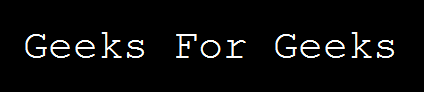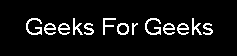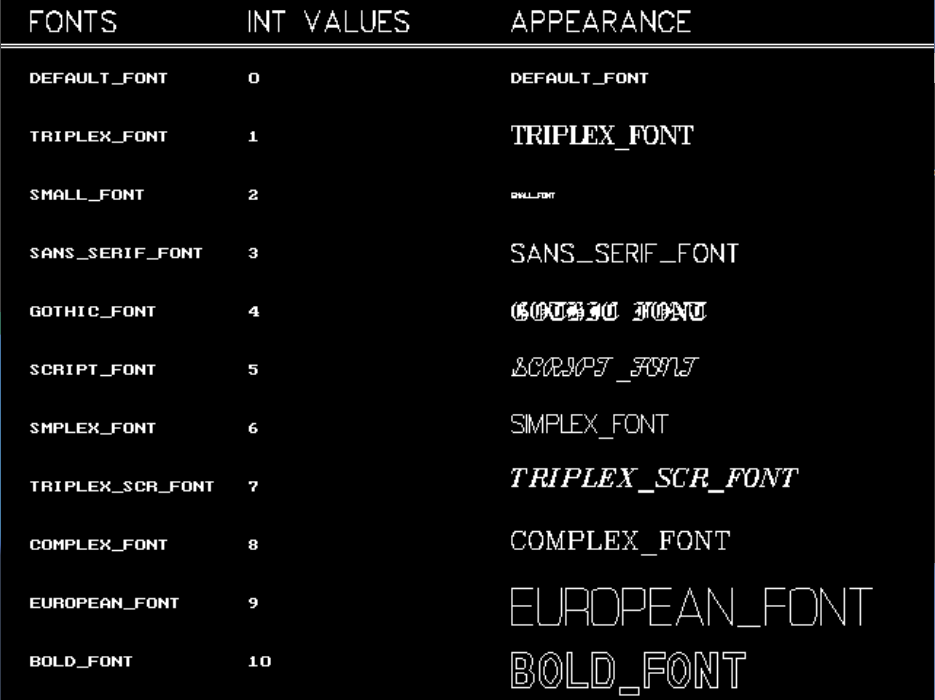# settextstyle function in C

• Difficulty Level : Basic
• Last Updated : 01 Dec, 2021

The header file graphics.h contains settextstyle() function which is used to change the way in which text appears. Using it we can modify the size of text, change direction of text and change the font of text.
Syntax :

```void settextstyle(int font, int direction, int font_size);

where,
font argument specifies the font of text,
Direction can be HORIZ_DIR (Left to right)
or VERT_DIR (Bottom to top).```

Examples :

```Input : font = 8, direction = 0, font_size = 5
Output : ``````Input : font = 3, direction = 0, font_size = 5
Output : ```The table below shows the fonts with their INT values and appearance:Below is the implementation of settextstyle() function :

## CPP

 `// C++ implementation for``// settextstyle() function``#include ` `// driver code``int` `main()``{``    ``// gm is Graphics mode which is``    ``// a computer display mode that``    ``// generates image using pixels.``    ``// DETECT is a macro defined in``    ``// "graphics.h" header file``    ``int` `gd = DETECT, gm;` `    ``// initgraph initializes the``    ``// graphics system by loading``    ``// a graphics driver from disk``    ``initgraph(&gd, &gm, ``""``);` `    ``// location of text``    ``int` `x = 150;``    ``int` `y = 150;` `    ``// font style``    ``int` `font = 8;` `    ``// font direction``    ``int` `direction = 0;` `    ``// font size``    ``int` `font_size = 5;` `    ``// for setting text style``    ``settextstyle(font, direction, font_size);` `    ``// for printing text in graphics window``    ``outtextxy(x, y, ``"Geeks For Geeks"``);` `    ``getch();``    ` `    ``// closegraph function closes the``    ``// graphics mode and deallocates``    ``// all memory allocated by graphics``    ``// system .``    ``closegraph();``    ` `    ``return` `0;``}`

Output:My Personal Notes arrow_drop_up